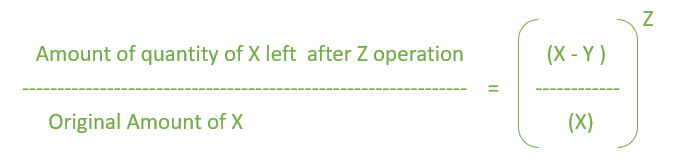# Program to find the quantity after mixture replacement

Given a container that has X liters of milk. Y liters of milk is drawn out and replaced with Y liters of water. This operation is done Z times. The task is to find out the quantity of milk left in the container.

Examples:

```Input: X = 10 liters, Y = 2 liters, Z = 2 times
Output: 6.4 liters

Input: X = 25 liters, Y = 6 liters, Z = 3 times
Output: 10.97 liters
```

## Recommended: Please try your approach on {IDE} first, before moving on to the solution.

Formula:-Below is the required implementation:

## C++

 `// C++ implementation using above formula ` `#include ` `using` `namespace` `std; ` ` `  `// Function to calculate the Remaining amount. ` `float` `Mixture(``int` `X, ``int` `Y, ``int` `Z) ` `{ ` `    ``float` `result = 0.0, result1 = 0.0; ` ` `  `    ``// calculate Right hand Side(RHS). ` `    ``result1 = ((X - Y) / (``float``)X); ` `    ``result = ``pow``(result1, Z); ` ` `  `    ``// calculate Amount left by ` `    ``// multiply it with original value. ` `    ``result = result * X; ` ` `  `    ``return` `result; ` `} ` ` `  `// Driver Code ` `int` `main() ` `{ ` `    ``int` `X = 10, Y = 2, Z = 2; ` ` `  `    ``cout << Mixture(X, Y, Z) << ``" litres"``; ` `    ``return` `0; ` `} `

## Java

 `// Java code using above Formula. ` `import` `java.io.*; ` ` `  `class` `GFG ` `{ ` `// Function to calculate the  ` `// Remaining amount.  ` `static` `double` `Mixture(``int` `X, ``int` `Y, ``int` `Z)  ` `{  ` `    ``double` `result1 = ``0.0``, result = ``0.0``;  ` ` `  `    ``// calculate Right hand Side(RHS).  ` `    ``result1 = ((X - Y) / (``float``)X);  ` `    ``result = Math.pow(result1, Z);  ` ` `  `    ``// calculate Amount left by  ` `    ``// multiply it with original value.  ` `    ``result = result * X;  ` ` `  `    ``return` `result;  ` `}  ` ` `  `// Driver Code ` `public` `static` `void` `main(String[] args) ` `{ ` `    ``int` `X = ``10``, Y = ``2``, Z = ``2``;  ` ` `  `    ``System.out.println((``float``)Mixture(X, Y, Z) +  ` `                                     ``" litres"``); ` `} ` `} ` ` `  `// This code is contributed  ` `// by Naman_Garg `

## Python 3

 `# Python 3 implementation using  ` `# above formula ` ` `  `# Function to calculate the ` `# Remaining amount. ` `def` `Mixture(X, Y, Z): ` ` `  `    ``result ``=` `0.0` `    ``result1 ``=` `0.0` ` `  `    ``# calculate Right hand Side(RHS). ` `    ``result1 ``=` `((X ``-` `Y) ``/` `X) ` `    ``result ``=` `pow``(result1, Z) ` ` `  `    ``# calculate Amount left by ` `    ``# multiply it with original value. ` `    ``result ``=` `result ``*` `X ` ` `  `    ``return` `result ` ` `  `# Driver Code ` `if` `__name__ ``=``=` `"__main__"``: ` `    ``X ``=` `10` `    ``Y ``=` `2` `    ``Z ``=` `2` ` `  `    ``print``(``"{:.1f}"``.``format``(Mixture(X, Y, Z)) ``+`  `                                   ``" litres"``) ` ` `  `# This code is contributed by ChitraNayal `

## C#

 `// C# code using above Formula. ` `using` `System; ` ` `  `class` `GFG ` `{ ` `// Function to calculate the  ` `// Remaining amount.  ` `static` `double` `Mixture(``int` `X,  ` `                      ``int` `Y, ``int` `Z)  ` `{  ` `    ``double` `result1 = 0.0, result = 0.0;  ` ` `  `    ``// calculate Right hand Side(RHS).  ` `    ``result1 = ((X - Y) / (``float``)X);  ` `    ``result = Math.Pow(result1, Z);  ` ` `  `    ``// calculate Amount left by  ` `    ``// multiply it with original value.  ` `    ``result = result * X;  ` ` `  `    ``return` `result;  ` `}  ` ` `  `// Driver Code ` `public` `static` `void` `Main() ` `{ ` `    ``int` `X = 10, Y = 2, Z = 2;  ` ` `  `    ``Console.WriteLine((``float``)Mixture(X, Y, Z) +  ` `                                    ``" litres"``); ` `} ` `} ` ` `  `// This code is contributed  ` `// by Akanksha Rai(Abby_akku) `

## PHP

 `  `

Output:

```6.4 litres
```

Attention reader! Don’t stop learning now. Get hold of all the important DSA concepts with the DSA Self Paced Course at a student-friendly price and become industry ready.

My Personal Notes arrow_drop_upCheck out this Author's contributed articles.

If you like GeeksforGeeks and would like to contribute, you can also write an article using contribute.geeksforgeeks.org or mail your article to contribute@geeksforgeeks.org. See your article appearing on the GeeksforGeeks main page and help other Geeks.

Please Improve this article if you find anything incorrect by clicking on the "Improve Article" button below.